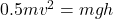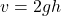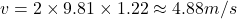(II) A sled is initially given a shove up a frictionless 23.0° incline. It reaches a maximum vertical height 1.22 m higher than where it sta

Question

(II) A sled is initially given a shove up a frictionless 23.0° incline. It reaches a maximum vertical height 1.22 m higher than where it started at the bottom. What was its initial speed?

in progress 0
5 months 2021-08-26T01:22:21+00:00 1 Answers 19 views 0

Using the law of conservation of energy, we know that energy can neither be created nor destroyed but can only be transformed from one state to another. Therefore, the kinetic energy at the inclined bottom equals to the potential energy at the top henceand making v the subject of the formula thenwhere m represnt the mass of sled, v is the initial speed, g is acceleration due to gravity and h ia the vertical height. Substituting g with 9.81 m/s2 and h with 1.22 then